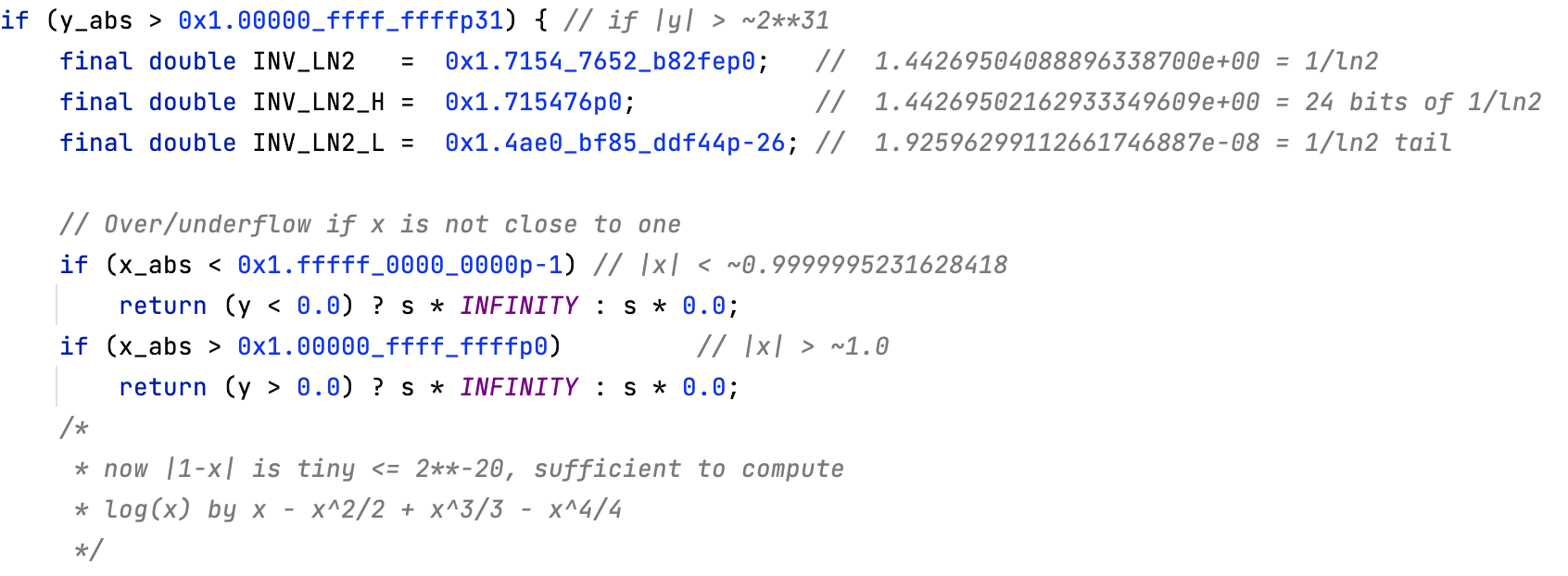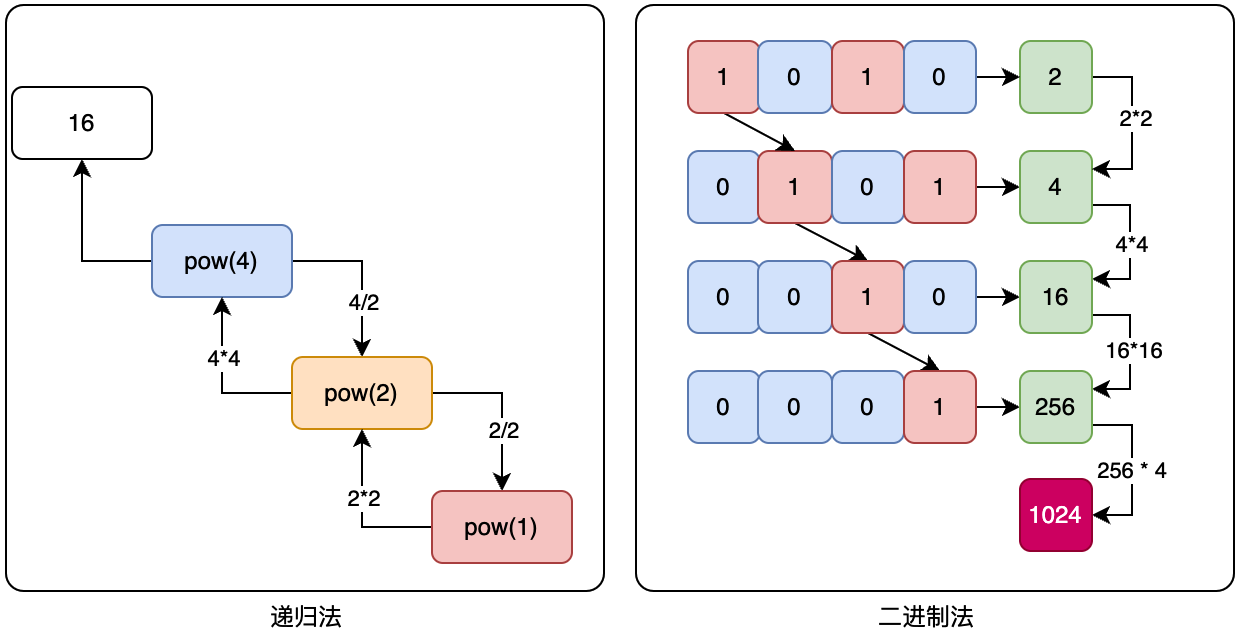# # 《程序员数学：快速计算次方》—— Math.pow() 函数源码如何实现的？

## # 一、前言## # 二、算法实现• 这里举例了2^4次幂递归计算和2^10次幂使用二进制移位。
• 接下来我们可以看下具体的代码实现。

### # 1. 递归

``````public static double pow01(double base, double power) {
if (power == 0) {
return 1;
}
if (power % 2 == 0) {
double multiplier = pow01(base, power / 2);
return multiplier * multiplier;
}
double multiplier = pow01(base, Math.floor(power / 2));
return multiplier * multiplier * base;
}
``````
1
2
3
4
5
6
7
8
9
10
11
• 把次方数不断的通过除2递归，计算乘积值。就和上图中的左面部分逻辑一致。

### # 2. 滑动窗口

``````public static long pow03(int base, int exponent) {
if (exponent == 0) {
return 1;
}
if (exponent == 1) {
return base;
}
long result = 1;
long window = base;
while (exponent > 0) {
if ((exponent & 1) == 1) {
result *= window;
}
window *= window;
exponent >>= 1;
}
return result;
}
``````
1
2
3
4
5
6
7
8
9
10
11
12
13
14
15
16
17
18
• 滑动窗口法是一种用于在一个数列中查找满足某些条件的子序列的算法。它的基本思路是，使用一个指针指向子序列的左端点，然后通过不断移动这个指针来扩展子序列的右端点，直到找到满足条件的子序列为止。

### # 3. 蒙哥马利的梯子技术

``````public static BigInteger pow04(BigInteger x, BigInteger n) {
BigInteger x1 = x;
BigInteger x2 = x.multiply(x);
for (int i = n.bitLength() - 2; i >= 0; i--) {
if (n.testBit(i)) {
x1 = x1.multiply(x2);
x2 = x2.multiply(x2);
} else {
x2 = x1.multiply(x2);
x1 = x1.multiply(x1);
}
}
return x1;
}
``````
1
2
3
4
5
6
7
8
9
10
11
12
13
14
• 蒙哥马利的梯子技术需要使用 BigInteger 类型的数据进行计算。BigInteger 类是 Java 中的一个用于处理任意精度整数的类。

### # 4. 固定底数

``````public static BigInteger pow05(BigInteger base, BigInteger exponent) {
int e = exponent.intValue();
BigInteger result = BigInteger.ONE;
BigInteger current = base;
while (e > 0) {
if ((e & 1) == 1) {
result = result.multiply(current);
}
current = current.multiply(current);
e >>= 1;
}
return result;
}
``````
1
2
3
4
5
6
7
8
9
10
11
12
13
• 固定底数指数法（Fixed-base exponentiation）是一种用于快速计算幂次方的算法。它的基本思路是使用预先计算的幂的表来减少求幂的次数。

## # 三、测试验证

``````@Test
public void test_FastPowering() {
System.out.println("测试结果：" + FastPowering.pow01(2, 4));
System.out.println("测试结果：" + FastPowering.pow02(2, 10));
System.out.println("测试结果：" + FastPowering.pow03(2, 4));
System.out.println("测试结果：" + FastPowering.pow04(BigInteger.valueOf(2), BigInteger.valueOf(10)));
System.out.println("测试结果：" + FastPowering.pow05(BigInteger.valueOf(2), BigInteger.valueOf(10)));
}
``````
1
2
3
4
5
6
7
8

``````测试结果：16.0

Process finished with exit code 0
``````
1
2
3
4
5
6
7